[ Home > Magnetic field from a 1m x 2.6m rectangular coil using ground plane as a conductor ]

# Magnetic field from a 1m x 2.6m rectangular coil using ground plane as a conductor

Note: these graphs show calculated Z-axis (perpendicular to the radiating coil in X-Y plane) components of magnetic field strength, relative to the value at the center of the coil.

## In a plane parallel to the radiating coil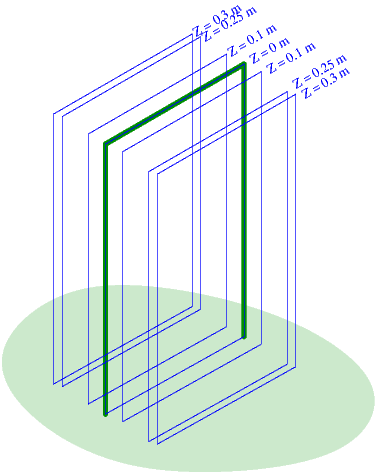Z = 0m Z = 0.1m Z = 0.25m Z = 0.3m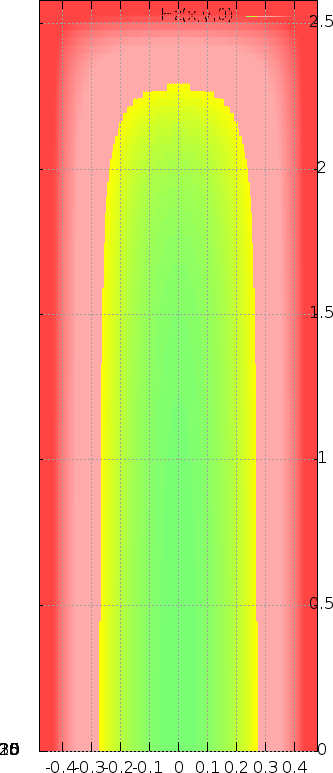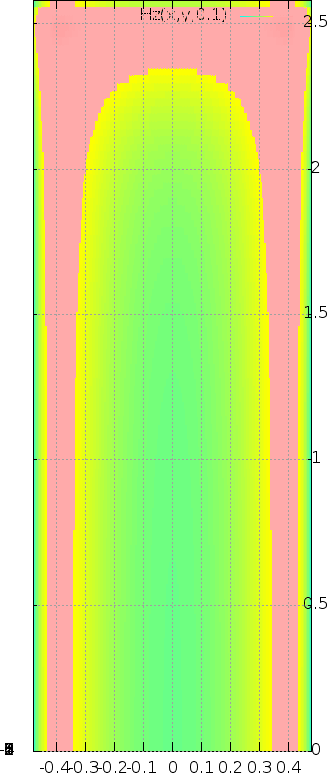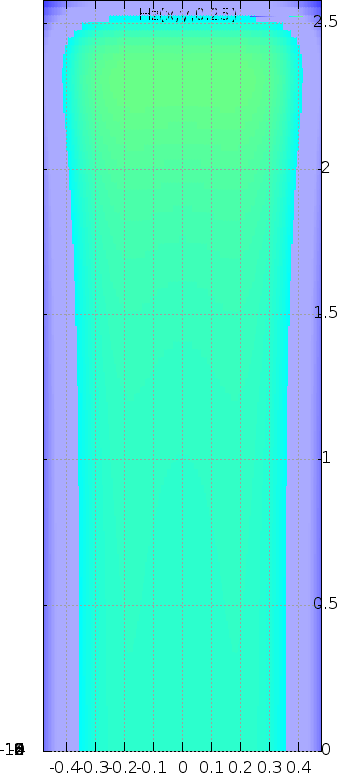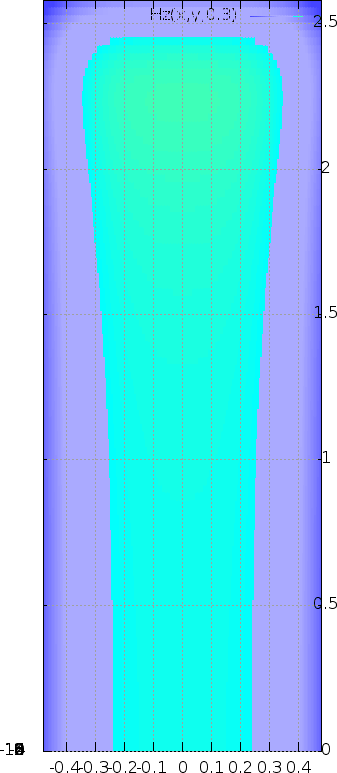## In a plane perpendicular to the radiating coil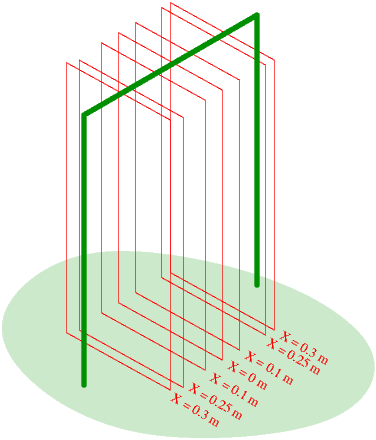X = 0m X = 0.1m X = 0.25m X = 0.3m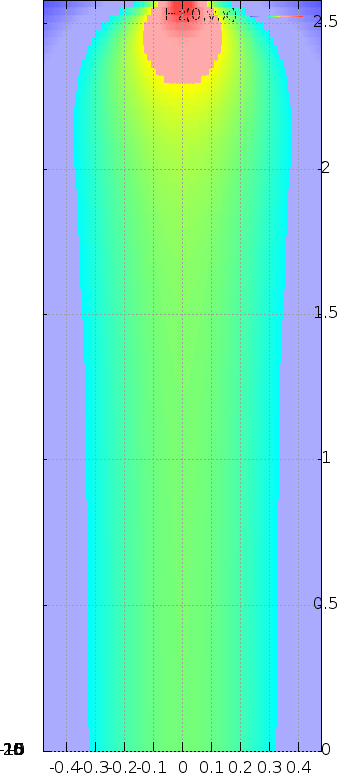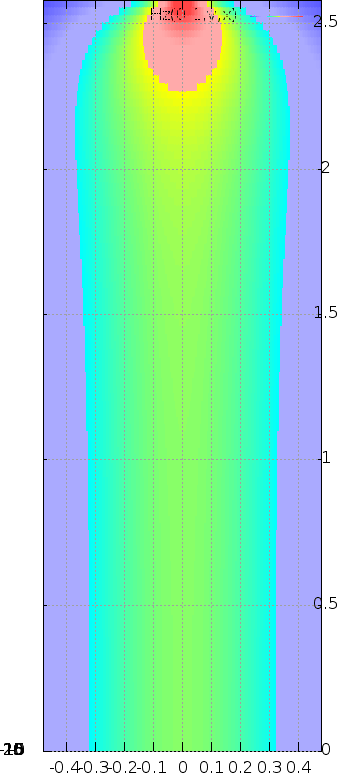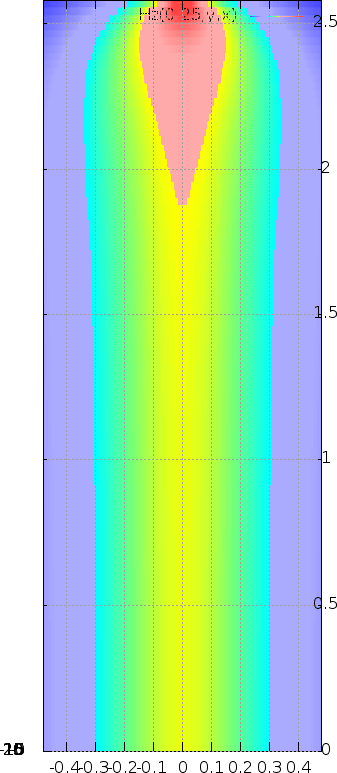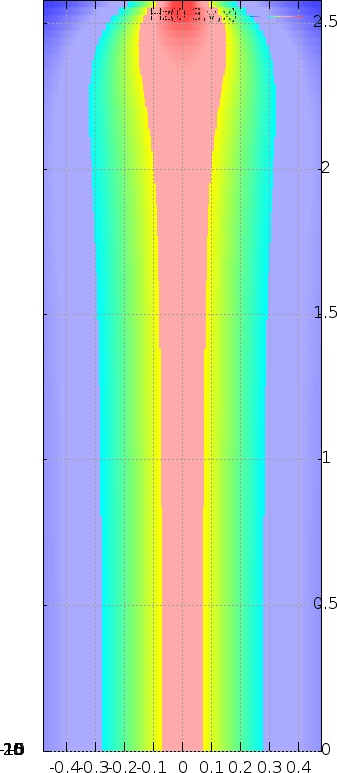2018-02-12, T.Sato